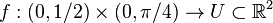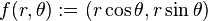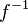# 1617-257/TUT-R-6

On 10/20/16, we discussed how one can calculate the derivative of the inverse of the map$f: (0,1/2) \times (0, \pi/4) \to U \subset \mathbb R^2$ where$f(r, \theta) := (r \cos \theta, r \sin \theta)$:
Method 1. Find$f^{-1}$ explicitly and differentiate it.